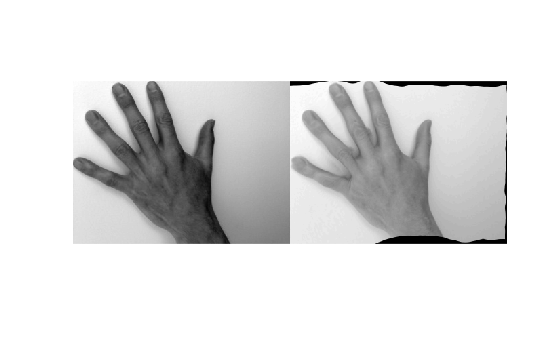# imregdemons

Estimate displacement field that aligns two 2-D or 3-D images

## Syntax

``````[D,moving_reg] = imregdemons(moving,fixed)``````
``[D,moving_reg] = imregdemons(moving,fixed,N)``
``[D,moving_reg] = imregdemons(___,Name,Value)``

## Description

example

``````[D,moving_reg] = imregdemons(moving,fixed)``` estimates the displacement field `D` that aligns the image to be registered, `moving`, with the reference image, `fixed`. The displacement vectors at each pixel location map locations from the `fixed` image grid to a corresponding location in the `moving` image. `moving_reg` is a warped version of the `moving` image that is warped according to the displacement field `D` and resampled using linear interpolation.```
````[D,moving_reg] = imregdemons(moving,fixed,N)` specifies the number of iterations to be computed. This function does not use a convergence criterion and therefore is always guaranteed to run for the specified or default number of iterations. ```

example

````[D,moving_reg] = imregdemons(___,Name,Value)` registers the moving image using name-value pairs to control aspects of weight computation.```

## Examples

collapse all

This example shows how to solve a registration problem in which the same hand has been photographed in two different poses. The misalignment of the images varies locally throughout each image. This is therefore a non-rigid registration problem.

Read the two images into the workspace.

```fixed = imread('hands1.jpg'); moving = imread('hands2.jpg');```

Convert the images to grayscale for processing.

```fixed = im2gray(fixed); moving = im2gray(moving);```

Observe the initial misalignment. Fingers are in different poses.

`imshowpair(fixed,moving,'montage')`Overlay the two images to make it easy to see where the images differ. The differences are highlighted in green and magenta.

`imshowpair(fixed,moving)`Correct illumination differences between the `moving` and `fixed` images using histogram matching. This is a common pre-processing step.

`moving = imhistmatch(moving,fixed);`

Estimate the transformation needed to bring the two images into alignment.

```[~,movingReg] = imregdemons(moving,fixed,[500 400 200],... 'AccumulatedFieldSmoothing',1.3);```

Display the results of the registration. In the first figure, the images are overlaid to show the alignment.

`imshowpair(fixed,movingReg)``imshowpair(fixed,movingReg,'montage')`## Input Arguments

collapse all

Image to be registered, specified as a 2-D or 3-D grayscale image.

Data Types: `single` | `double` | `int8` | `int16` | `int32` | `uint8` | `uint16` | `uint32` | `logical`

Reference image in the target orientation, specified as a 2-D or 3-D grayscale image.

Data Types: `single` | `double` | `int8` | `int16` | `int32` | `uint8` | `uint16` | `uint32` | `logical`

Number of iterations, specified as a positive integer scalar or vector.

When you specify a vector, `N` is the number of iterations per pyramid level (resolution level). For example, if there are 3 pyramid levels, then you can specify the vector `[100,50,25]`, where `imregdemons` performs 100 iterations at the lowest resolution level, 50 iterations at the next pyramid level, and 25 iterations at the last iteration level — the level with full resolution. Because it takes less time to process the lower resolution levels, running more iterations at low resolution and fewer iterations at the higher resolutions of the pyramid can help performance.

Data Types: `single` | `double` | `int8` | `int16` | `int32` | `uint8` | `uint16` | `uint32`

### Name-Value Arguments

Specify optional comma-separated pairs of `Name,Value` arguments. `Name` is the argument name and `Value` is the corresponding value. `Name` must appear inside quotes. You can specify several name and value pair arguments in any order as `Name1,Value1,...,NameN,ValueN`.

Example: `'AccumulatedFieldSmoothing',1.5` applies Gaussian smoothing with a standard deviation of 1.5 at each iteration

Smoothing applied at each iteration, specified as the comma-separated pair consisting of `'AccumulatedFieldSmoothing'` and a numeric value. This parameter controls the amount of diffusion-like regularization. `imregdemons` applies the standard deviation of the Gaussian smoothing to regularize the accumulated field at each iteration. Larger values result in smoother output displacement fields. Smaller values result in more localized deformation in the output displacement field. Values typically are in the range [0.5, 3.0]. When you specify multiple `PyramidLevels`, the standard deviation used in the Gaussian smoothing remains the same at each pyramid level.

Data Types: `double`

Number of multi-resolution image pyramid levels to use, specified as the comma-separated pair consisting of `'PyramidLevels'` and a positive integer.

Data Types: `double`

Display wait bar to indicate progress, specified as the comma-separated pair consisting of `'DisplayWaitbar'` and the value `true` or `false`. When set to `true`, `imregdemons` displays a wait bar to indicate progress for long-running operations. To prevent `imregdemons` from displaying a wait bar, set `DisplayWaitbar` to `false`.

Data Types: `single` | `double` | `int8` | `int16` | `int32` | `int64` | `uint8` | `uint16` | `uint32` | `uint64` | `logical`

## Output Arguments

collapse all

Displacement field, specified as a numeric array. Displacement values are in units of pixels.

• If `fixed` is a 2-D grayscale image of size m-by-n, then the displacement field array is m-by-n-by-2. `D(:,:,1)` contains displacements along the x-axis and `D(:,:,2)` contains displacements along the y-axis.

• If `fixed` is a 3-D grayscale image of size m-by-n-by-p, then the displacement field array is m-by-n-by-p-by-3. `D(:,:,:,1)` contains displacements along the x-axis, `D(:,:,:,2)` contains displacements along the y-axis, and `D(:,:,:,3)` contains displacements along the z-axis.

Data Types: `double`

Registered image, returned as a 2-D or 3-D grayscale image. The image is warped according to the displacement field `D` and resampled using linear interpolation.

## Tips

 Thirion, J.-P. "Image matching as a diffusion process: an analogy with Maxwell’s demons". Medical Image Analysis. Vol. 2, Number 3, 1998, pp. 243–260.

 Vercauteren, T., X. Pennec, A. Perchant, N. Ayache, "Diffeomorphic Demons: Efficient Non-parametric Image Registration", NeuroImage. Vol. 45, Number 1, Supplement 1, March 2009, pp. 61–72.